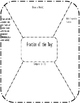# Fraction of the DaySubject
Resource Type
File Type
PDF (95 KB|2 pages)
Standards
\$1.50
• Product Description
• Standards
This packet is for students to work with fractions on a daily basis, covering several concepts of fractions (Mostly 4th grade standards).
Apply and extend previous understandings of multiplication to multiply a fraction or whole number by a fraction.
Apply and extend previous understandings of multiplication to multiply a fraction by a whole number.
Understand a fraction 𝘢/𝘣 with 𝘢 > 1 as a sum of fractions 1/𝘣.
Compare two fractions with different numerators and different denominators, e.g., by creating common denominators or numerators, or by comparing to a benchmark fraction such as 1/2. Recognize that comparisons are valid only when the two fractions refer to the same whole. Record the results of comparisons with symbols >, =, or <, and justify the conclusions, e.g., by using a visual fraction model.
Explain why a fraction 𝘢/𝘣 is equivalent to a fraction (𝘯 × 𝘢)/(𝘯 × 𝘣) by using visual fraction models, with attention to how the number and size of the parts differ even though the two fractions themselves are the same size. Use this principle to recognize and generate equivalent fractions.
Total Pages
2 pages